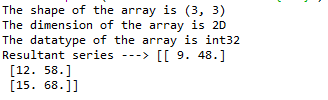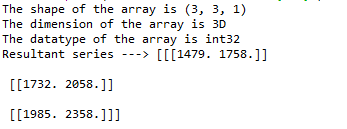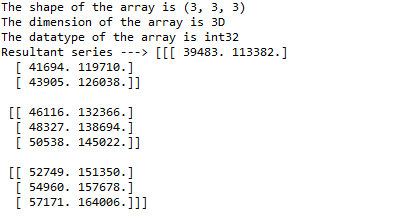# Python Program to Evaluate a Chebyshev Series at Points X When Coefficients are multi-dimensional

• Last Updated : 04 May, 2022

In this article, we will discuss how to evaluate a Chebyshev Series at points X when coefficients are multi-dimensional.

To evaluate the Chebyshev series at points, NumPy provides a function called chebyshev.chebval which can be used to integrate the Chebyshev series.

Syntax: Chebyshev.chebval(x, c, tensor)

Parameters:

• x – array_like, compatible object. If x is a list or tuple, it is converted to an array, otherwise, it is left unchanged and treated as a scalar. In either case, x or its elements must support addition and multiplication with themselves and with the elements of c.
• c – array_like. An array of coefficients is ordered so that the coefficients for terms of degree n are contained in c[n]. If c is multidimensional the remaining indices enumerate multiple polynomials. In the two-dimensional case, the coefficients may be thought of as stored in the columns of c.
• tensor – boolean. If True, the shape of the coefficient array is extended with ones on the right, one for each dimension of x.

### Example 1:

In the first example. let us consider a 2D array and evaluate it in the point [1,2]. Import the necessary packages as shown and pass the appropriate parameters as shown below.

## Python3

 `import` `numpy as np``from` `numpy.polynomial ``import` `chebyshev` `# multidimensional array of coefficients``c ``=` `np.arange(``9``).reshape(``3``, ``3``)` `print``(f``'The shape of the array is {c.shape}'``)``print``(f``'The dimension of the array is {c.ndim}D'``)``print``(f``'The datatype of the array is {c.dtype}'``)` `# pass the points to evaluate at x to the``# chebval function``res ``=` `chebyshev.chebval([``1``, ``2``], c, tensor``=``True``)` `# chebyshev series evaluated at point [1,2]``print``(f``'Resultant series ---> {res}'``)`

Output:### Example 2:

In the first example. let us consider a 3D array and evaluate it in the point [11,12]. Import the necessary packages as shown and pass the appropriate parameters as shown below.

## Python3

 `import` `numpy as np``from` `numpy.polynomial ``import` `chebyshev` `# multidimensional array of coefficients``c ``=` `np.arange(``9``).reshape(``3``, ``3``, ``1``)` `print``(f``'The shape of the array is {c.shape}'``)``print``(f``'The dimension of the array is {c.ndim}D'``)``print``(f``'The datatype of the array is {c.dtype}'``)` `# pass the points to evaluate at x to the chebval function``res ``=` `chebyshev.chebval([``11``, ``12``], c, tensor``=``True``)` `# chebyshev series evaluated at point [1,2]``print``(f``'Resultant series ---> {res}'``)`

Output:Output

### Example 3:

In the third example. let us consider a different 3D array of shape (3,3,3) and evaluate it in the point [33,56]. Import the necessary packages as shown and pass the appropriate parameters as shown below.

## Python3

 `import` `numpy as np``from` `numpy.polynomial ``import` `chebyshev` `# multidimensional array of coefficients``c ``=` `np.arange(``27``).reshape(``3``, ``3``, ``3``)` `print``(f``'The shape of the array is {c.shape}'``)``print``(f``'The dimension of the array is {c.ndim}D'``)``print``(f``'The datatype of the array is {c.dtype}'``)` `# pass the points to evaluate at x to the chebval function``res ``=` `chebyshev.chebval([``33``, ``56``], c, tensor``=``True``)` `# chebyshev series evaluated at point [33,56]``print``(f``'Resultant series ---> {res}'``)`

Output:My Personal Notes arrow_drop_up